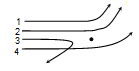In Bohr model of hydrogen atom, the ratio of periods of revolution of an electron in n =2 and n = 1 orbits is

(a) 2 : 1              (b) 4 : 1
(c) 8 : 1              (d) 16 : 1

Concept Questions :-

Bohr's model of atom
High Yielding Test Series + Question Bank - NEET 2020

Difficulty Level:

The ratio of the longest to shortest wavelengths in Brackett series of hydrogen spectra is
(a) $\frac{25}{9}$            (b) $\frac{17}{6}$
(c) $\frac{9}{5}$              (d) $\frac{4}{3}$

Concept Questions :-

Spectral series
High Yielding Test Series + Question Bank - NEET 2020

Difficulty Level:

The electron in a hydrogen atom makes a transition from an excited state to the ground state. Which of the following statements is true
(a) Its kinetic energy increases and its potential and total energies decrease
(b) Its kinetic energy decreases, potential energy increases and its total energy remains the same
(c) Its kinetic and total energies decrease and its potential energy increases
(d) Its kinetic, potential and total energies decreases

Concept Questions :-

Spectral series
High Yielding Test Series + Question Bank - NEET 2020

Difficulty Level:

The ratio of minimum to maximum wavelength in Balmer series is
(a) 5 : 9            (b) 5 : 36
(c) 1 : 4            (d) 3 : 4

Concept Questions :-

Spectral series
High Yielding Test Series + Question Bank - NEET 2020

Difficulty Level:

Rutherford’s $\mathrm{\alpha }$-particle experiment showed that the atoms have
(a) Proton          (b) Nucleus
(c) Neutron        (d) Electrons

Concept Questions :-

Various atomic model
High Yielding Test Series + Question Bank - NEET 2020

Difficulty Level:

Which of the following is true for number of spectral lines in going form Layman series to Pfund series
(a) Increases
(b) Decreases
(c) Unchanged
(d) May decreases or increases

Concept Questions :-

Spectral series
High Yielding Test Series + Question Bank - NEET 2020

Difficulty Level:

Radius of the first orbit of the electron in a hydrogen atom is 0.53 Å. So, the radius of the third orbit will be

(a) 2.12 Å             (b) 4.77 Å
(c) 1.06 Å             (d) 1.59 Å

Concept Questions :-

Bohr's model of atom
High Yielding Test Series + Question Bank - NEET 2020

Difficulty Level:

The first line in the Lyman series has wavelength $\mathrm{\lambda }$. The wavelength of the first line in Balmer series is

(a) $\frac{2}{9}\mathrm{\lambda }$              (b) $\frac{9}{2}\mathrm{\lambda }$
(c) $\frac{5}{27}\mathrm{\lambda }$            (d) $\frac{27}{5}\mathrm{\lambda }$

Concept Questions :-

Spectral series
High Yielding Test Series + Question Bank - NEET 2020

Difficulty Level:

In the following transitions, which one has higher frequency

(a) 3 – 2              (b) 4 – 3
(c) 4 – 2              (d) 3 – 1

Concept Questions :-

Bohr's model of atom
High Yielding Test Series + Question Bank - NEET 2020

Difficulty Level:

The diagram shows the path of four $\alpha$-particles of the same energy being scattered by the nucleus of an atom simultaneously. Which of these are/is not physically possible?(a) 3 and 4            (b) 2 and 3
(c) 1 and 4            (d) 4 only

Concept Questions :-

Various atomic model